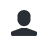# Chat Calculator 0.3

Plugin which allows players to perform basic maths calculations

1.Tested Minecraft Versions:
• 1.12Hi,

This is a simple plugin which allows players to perform basic maths calculations while on the server. Whether you need to calculate sums regarding your in-game economy or you just want to do your maths homework while playing! This plugin has got you covered.

Usage:
The plugin is very simple to use, here are the commands that you can use:
/cal <number>-<number> (subtract)
/cal <number>/<number> (divide)
/cal <number>*<number> (multiply)
/cal <number> power <number> (Calculates the 1st number to the power of the second number
/cal random <number> to <number> (Chooses a random number between these ranges)
/cal sqrt <number> (Calculates the square root of a number)
/cal setmem <number> (Sets memory of a number which can be re-called in any calculation using keyword 'mem')

You can now use keyword 'pi' anywhere in a calculation and it will give the value of pi.

Thanks, let me know of any improvements/fixes!MINSA20, Banjo226 and Rocknest like this.

1. Updated and tested on 1.12.2
3. Extra functions

### Recent Reviews

1.TheFroland
1/5,
Version: 0.3
I dont think there is a use for this plugin. WorldEdit has a inbuilt calc which can do a lot more than this! But its great for people without WorldEdit
2.ProxyBite
5/5,
Version: 0.2
Excelent idea
3.Janhektor
5/5,
Version: 2015-03-31
Nice idea!
Can you add commands/subcommands to perform more complex calculations? Like areas and volumns of geometric figures or functions for power/root.
1.Author's Response
Sounds like a good idea! Thanks, I will start to introduce more complex commands! :D
4.Shark998
4/5,
Version: 2015-03-31
Awesome idea! My players gonna love this! :) But I think that more features are needed.. like a config for messages, or a feature that allows you to set your own symbol for calculations (like "x" for multiply, or ":" for divide)
Also, with more calculations, this plugin will be more better :P
1.Author's Response